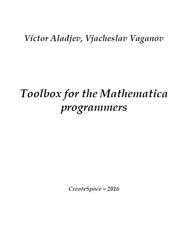# Toolbox for the Mathematica programmers, Aladjev V., Vaganov V., 2016

Toolbox for the Mathematica programmers, Aladjev V., Vaganov V., 2016.

Systems of computer mathematics find more and more broad application in a number of natural, economical and social fields. These systems are rather important tools for scientists, teachers, researchers and engineers, very well combining symbolical methods with advanced computing methods. One of leaders among tools of this class undoubtedly is the Mathematica system. The book focuses on a important aspect – modular programming supported by Mathematica. The given aspect is of particular importance not only for appendices but also above all it is quite important in the creation of the user tools that expand the most frequently used standard tools of the system and/or eliminate its shortcomings, or complement the new facilities.Headings of the user procedures and functions in the Mathematica system.
In many contexts, it is not necessary to know the exact value of an arbitrary expression; it suffices to know that the given expression belongs to a certain broad class, or group, of expressions which share some common properties. These classes or groups are known as types. If T represents a type, then an expression is of type T if it belongs to the class that T presents. For example, an expression is said to be of type Integer if it belongs to the definite class of expressions denoted by the type name Integer, which is the set of integers. Many procedures and functions use the types to direct the flow of control in algorithms or to decide whether an expression is a valid input. For example, the behavior of a function or a procedure generally depends on the types of its actual arguments. At that, the result of a number of operations is defined by type of their arguments. The type – fundamental concept of the theory of programming, defining an admissible set of values or operations which can be applied to such values and, perhaps, also a way of realization of storage of values and performance of operations.

Contents.
Preface.
Chapter 1.Additional tools in interactive mode of the Mathematica.
Chapter 2.Additional tools of processing of expressions in the Mathematica software.
Chapter 3.Additional tools of processing of symbols and string structures in the Mathematica system.
Chapter 4.Additional tools of processing of sequences and lists in the Mathematica software.
Chapter 5.The additional tools expanding the standard Mathematica functions, or its software as a whole.
Chapter 6.Problems of procedural programming in the Mathematica software.
Chapter 7.Software for input–output in the Mathematica system.
Chapter 8.The manipulations organization with the user packages in the Mathematica software.
References.
About the Authors.

Бесплатно скачать электронную книгу в удобном формате, смотреть и читать:
Скачать книгу Toolbox for the Mathematica programmers, Aladjev V., Vaganov V., 2016 - fileskachat.com, быстрое и бесплатное скачивание.

Скачать zip
Ниже можно купить эту книгу по лучшей цене со скидкой с доставкой по всей России.Купить эту книгу

Скачать - zip,pdf - Яндекс.Диск.
Дата публикации:

Теги: :: :: :: :: :: ::